Complex Fractions: More Examples (page 2 of 2)• Simplify the following expression:
•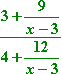Can I start by hacking off the x – 3's? Can I cancel the 4 with the 12? Or the 3 with the 9 or the 12? (Hint: No!)

 The common denominator for this complex fraction would be x – 3, so I'll multiply through, top and bottom, by that.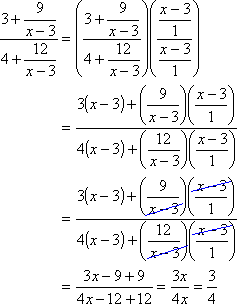Clearly, nothing cancels, so my final answer is: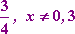It is highly unusual for a complex fraction to simplify this much, but it can happen. In this case, the "except for x equal to 3" part is rather important, since the original fraction is not always equal to 3/4. Indeed, it is not even defined for x equal to 3 (since this would cause division by zero).

• Simplify the following expression:
•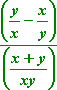Can I start off by canceling like this:

 DON'T DO THIS!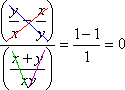I can only cancel factors, not terms, so the above cancellations are not proper.

 The first thing I need to do is multiply through, top and bottom, by the common denominator of xy.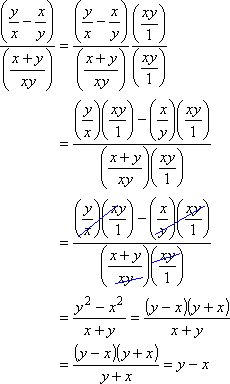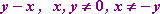(Why the restrictions? )

• Simplify the following expression:
•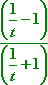Can I start by canceling off the 1's or the 1/t's? (Hint: No!)

 I'll multiply through, top and bottom, by the common denominator of t.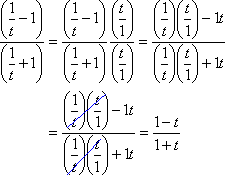Can I cancel off the t's now? Or cancel off the 1's? (Hint: No!) I can only cancel off factors, not terms, and nothing factors here, so this is as simplified as it gets. The final answer is: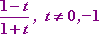(Why the restrictions? )

When working with complex fractions, be careful to show each step completely. Don't try to skip steps or do everything in your head. And don't get careless with cancellation; remember that you can only cancel factors, not terms. If you remember this, and do your work clearly, you should be fairly successful with these problems.